# CSS | Styling Forms

CSS form is used to create interactive form for user. It provides many ways to set the style.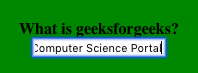There are many CSS properties available which can be used to create and style HTML forms to make them more interactive, some of which are listed below:

• Attribute Selector: The attribute type of the input form can take a variety of form depending on user’s choice. It could be anything out of the possible types like text, search, url, tel, email, password, date pickers, number, checkbox, radio, file etc. User needs to specify type while creating a form.

Example:

 ` ` `<``html``> ` `    ``<``head``> ` `        ``<``style``> ` `        ``body{ ` `            ``background-color:green; ` `        ``} ` `        `` ` `    `` ` ` `  `    ``<``body``> ` `        ``<``center``> ` `            ``<``b``>Is Geeksforgeeks useful ? ` `            ``<``form``> ` `                ``<``input` `type``=``"radio"` `name``=``"useful"` `value``=``"yes"` `checked>  ` `                ``Yes <``br``> ` `                ``<``input` `type``=``"radio"` `name``=``"useful"` `value``=``"def_yes"``>  ` `                ``Definitely Yes   ` `            `` ` `        `` ` `    `` ` `                     `

Output: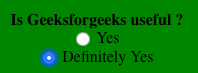Consider another example where the input type is simply a text:

 ` ` `<``html``> ` `<``head``> ` `    ``<``style``> ` `        ``body{ ` `            ``background-color:green; ` `        ``} ` `    `` ` ` ` ` `  `<``body``> ` `    ``<``center``> ` `    ``<``form``> ` `        ``<``b``>Do you find Geeksforgeeks helpful?    ` `            ``<``br``> ` `        ``<``input` `type``=``"text"` `name``=``"info"``><``br``> ` `    `` ` `    `` ` ` ` `                     `

Output: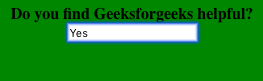• Styling the Width of Input: The width property is used to set the width of the input field. Consider the below example where the width is set to be 10% of the entire screen.

 ` ` `<``html``> ` `<``head``> ` `    ``<``style``> ` `        ``input{ ` `            ``width:10%; ` `        ``} ` `         `  `        ``body{ ` `            ``background-color:green; ` `        ``} ` `    `` ` ` ` ` `  `<``body``> ` `    ``<``center``> ` `    ``<``form``> ` `        ``<``b``>Do you find Geeksforgeeks helpful? ` `            ``<``br``> ` `        ``<``input` `type``=``"text"` `name``=``"info"``><``br``> ` `    `` ` `    `` ` ` ` `                     `

Output: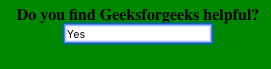` ` `<``html``> ` `<``head``> ` `    ``<``style``> ` `        ``input{ ` `            ``width:10%; ` `            ``padding: 12px; ` `        ``} ` `         `  `        ``body{ ` `            ``background-color:green; ` `        ``} ` `    `` ` ` ` ` `  `<``body``> ` `    ``<``center``> ` `        ``<``form``> ` `            ``<``b``>Do you find Geeksforgeeks helpful?<``br``> ` `            ``<``input` `type``=``"text"` `name``=``"info"``><``br``> ` `        `` ` `    `` ` ` ` `                     `

Output: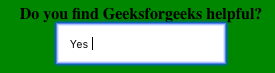• Set Margin for Inputs: The margin property is used to add space outside the input field. It is helpful when there are many inputs. Consider the example below with two inputs and observe the space (margin) between them.

 ` ` `<``html``> ` `<``head``> ` `    ``<``style``> ` `        ``input{ ` `            ``width:10%; ` `            ``margin: 8px; ` `        ``} ` `         `  `        ``body{ ` `            ``background-color:green; ` `        ``} ` `    `` ` ` ` ` `  `<``body``> ` `    ``<``center``> ` `    ``<``form``> ` `        ``<``b``>Mention two topics that you liked on Geeksforgeeks ` `            ``<``br``> ` `        ``<``input` `type``=``"text"` `name``=``"info"``><``br``> ` `        ``<``input` `type``=``"text"` `name``=``"info"``><``br``> ` `    `` ` `    `` ` ` ` `                     `

Output: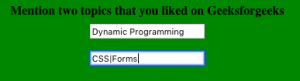• Adding Border and Border-radius: The border property is used to bring change in the size and color of the border whereas border-radius property is used for adding rounded corners.

Consider the below example where a 2px solid red border is created with a border radius of 4px.

 ` ` `<``html``> ` `<``head``> ` `    ``<``style``> ` `        ``input{ ` `            ``width:10%; ` `            ``margin: 8px; ` `            ``border: 2px solid red; ` `            ``border-radius: 4px; ` `        ``} ` `         `  `        ``body{ ` `            ``background-color:green; ` `        ``} ` `    `` ` ` ` ` `  `<``body``> ` `    ``<``center``> ` `        ``<``form``> ` `            ``<``b``> ` `                ``Mention two topics that you liked on  ` `                ``Geeksforgeeks ` `            `` ` `            ``<``br``> ` `            ``<``input` `type``=``"text"` `name``=``"info"``><``br``> ` `            ``<``input` `type``=``"text"` `name``=``"info"``><``br``> ` `        `` ` `    `` ` ` ` `                     `

Output: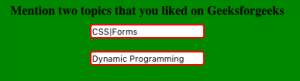Note: User can also have border at any particular side and remove others or have all borders of different color. Consider the below example where user want border only on top(blue color) and bottom(red color).

 ` ` `<``html``> ` `<``head``> ` `    ``<``style``> ` `        ``input{ ` `            ``width:10%; ` `            ``margin: 8px; ` `            ``border: none; ` `            ``border-top: 3px solid blue; ` `            ``border-bottom: 3px solid red; ` `        ``} ` `         `  `        ``body{ ` `            ``background-color:green; ` `        ``} ` `    `` ` ` ` ` `  `<``body``> ` `    ``<``center``> ` `        ``<``form``> ` `            ``<``b``> ` `                ``Mention two topics that you liked on  ` `                ``Geeksforgeeks ` `            `` ` `            ``<``br``> ` `            ``<``input` `type``=``"text"` `name``=``"info"``><``br``> ` `            ``<``input` `type``=``"text"` `name``=``"info"``><``br``> ` `        `` ` `    `` ` ` ` `                     `

Output: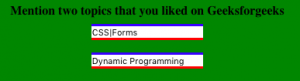• Adding Color to text and Background: The color property is used to change the color of the text in the input and the background-color property is used to change the color of the background of the input field.

Consider the below example where color of text is black and background color is set to green.

 ` ` `<``html``> ` `<``head``> ` `    ``<``style``> ` `        ``input{ ` `            ``width:10%; ` `            ``margin: 8px; ` `            ``border: none; ` `            ``background-color: green; ` `            ``color: black; ` `        ``} ` `         `  `        ``body{ ` `            ``background-color:white; ` `        ``} ` `    `` ` ` ` ` `  `<``body``> ` `    ``<``center``> ` `        ``<``form``> ` `            ``<``b``> ` `                ``Mention two topics that you liked  ` `                ``on Geeksforgeeks ` `            `` ` `            ``<``br``> ` `            ``<``input` `type``=``"text"` `name``=``"info"``><``br``> ` `            ``<``input` `type``=``"text"` `name``=``"info"``><``br``> ` `        `` ` `    `` ` ` ` `                     `

Output: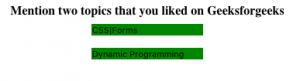• Focus Selector: When we click on the input field it gets an outline of blue color. You can change this behaviour by using :focus selector.

Consider the below example where user wants a 3px solid red outline and green background when focused.

 ` ` `<``html``> ` `<``head``> ` `    ``<``style``> ` `        ``input{ ` `            ``width:10%; ` `            ``margin: 8px; ` `            ``color: black; ` `        ``} ` `         `  `        ``input[type=text]:focus { ` `        ``border: 3px solid red; ` `        ``background-color: green; ` `        ``} ` `         `  `        ``body{ ` `            ``background-color:white; ` `        ``} ` `    `` ` ` ` ` `  `<``body``> ` `    ``<``center``> ` `    ``<``form``> ` `        ``<``b``> ` `            ``Mention two topics that you liked  ` `            ``on Geeksforgeeks ` `        `` ` `        ``<``br``> ` `        ``<``input` `type``=``"text"` `name``=``"info"``><``br``> ` `        ``<``input` `type``=``"text"` `name``=``"info"``><``br``> ` `    `` ` `    `` ` ` ` `                     `

Output: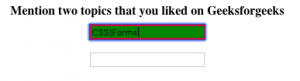• Adding images in the Input form: The background-image property can be used to put an image inside the input form and it can be positioned using background-position property and user can also decide whether to repeat or not.

Consider the example below with image in background with no-repeat mode.

 ` ` `<``html``> ` `<``head``> ` `    ``<``style``> ` `        ``input{ ` `            ``width: 20%; ` `            ``background-image: url('search.png'); ` `            ``background-position: 10px 10px;  ` `            ``background-repeat: no-repeat; ` `            ``padding: 12px 20px 12px 40px; ` `        ``} ` `         `  `        ``body{ ` `            ``background-color:white; ` `        ``} ` `    `` ` ` ` ` `  `<``body``> ` `    ``<``center``> ` `    ``<``form``> ` `        ``<``b``>Search on Geeksforgeeks<``br``> ` `        ``<``input` `type``=``"text"` `name``=``"info"` `placeholder``=``"Search.."``><``br``> ` `    `` ` `    `` ` ` ` `                     `

Output: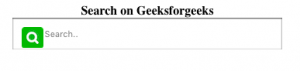• Transition Property: The transition property can be used over the input field to bring change in the size of the field by specifying the relaxed width and focused width along with the time period for which operation will take place.

Consider below example where relaxed input field width is 15% which when focused changes to 30% in 1 second.

 ` ` `<``html``> ` `<``head``> ` `    ``<``style``> ` `        ``input{ ` `            ``width: 15%; ` `            ``-webkit-transition: width 1s ease-in-out; ` `            ``transition: width 1s ease-in-out; ` `        ``} ` `         `  `        ``input[type=text]:focus { ` `            ``width: 30%; ` `            ``border:4px solid blue; ` `        ``} ` `         `  `        ``body{ ` `            ``background-color:green; ` `        ``} ` `    `` ` ` ` ` `  `<``body``> ` `    ``<``center``> ` `    ``<``form``> ` `        ``<``b``>Search on Geeksforgeeks<``br``> ` `        ``<``input` `type``=``"text"` `name``=``"info"` `placeholder``=``"Search.."``><``br``> ` `    `` ` `    `` ` ` ` `                     `

Output:
When Relaxed-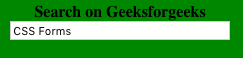When focused-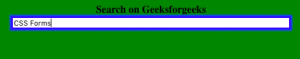My Personal Notes arrow_drop_upCheck out this Author's contributed articles.

If you like GeeksforGeeks and would like to contribute, you can also write an article using contribute.geeksforgeeks.org or mail your article to contribute@geeksforgeeks.org. See your article appearing on the GeeksforGeeks main page and help other Geeks.

Please Improve this article if you find anything incorrect by clicking on the "Improve Article" button below.

Article Tags :

4

Please write to us at contribute@geeksforgeeks.org to report any issue with the above content.